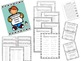# Mixed Timed Test BundleSubject
Resource Type
Format
Zip (3 MB|39 pages)
Standards
\$4.50
Bundle
List Price:
\$5.00
You Save:
\$0.50
Products in this Bundle (2)
1. This isn't just your ordinary old timed tests that only test one digit facts and then the skill is mastered. These tests build on each other in complexity of skills. Students will be challenged to add and subtract groups of ten, finding the missing number, and determining if both sides of the equal
2. This isn't just your ordinary old timed tests that only test one digit facts and then the skill is mastered. These tests build on each other in complexity of skills. Students will be challenged to multiply and divide basic facts on the same page, multiply and divide groups of ten, and find the missi
Also included in
1. This bundle includes my Addition, Subtraction, Mixed Addition and Subtraction, Multiplication, Division, and Mixed Multiplication and Division Timed Tests packs! These tests build on each other in complexity of skills. Students will be challenged to add, subtract, multiply, and divide basic facts, g
\$13.50
\$15.00
Save \$1.50
• Bundle Description
• Standards

This bundle includes two of my timed tests best sellers, Mixed Addition & Subtraction and Mixed Multiplication & Division, all into one! I noticed that people were buying these tests in the same transaction and I wanted to make it easier on the buyer!

These tests are unique in the way that students are required to solve addition & subtraction problems on the same timed test and multiplication & division problems on the same timed test. This helps students practice paying attention to what the sign is in the problem and how to switch between two different operations. These tests build on each other in complexity of skills. Students will be challenged to solve basic facts on the same page, add and subtract or multiply and divide groups of ten, and find the missing number. These tests have allowed my high students show their understanding of skills and my lower students see success and progress!

Each test has an answer key and there is also a chance for the students to keep track of their progress by completing a bar graph. There is also a certificate for completion of these timed tests.

If you like these timed tests, consider checking out my addition, subtraction, multiplication, and division timed tests and bundles! They are great for many different grades and learners!

*Don't forget to leave feedback and let me know how this worked in your classroom! Thank you for your business!

to see state-specific standards (only available in the US).
Fluently multiply and divide within 100, using strategies such as the relationship between multiplication and division (e.g., knowing that 8 × 5 = 40, one knows 40 ÷ 5 = 8) or properties of operations. By the end of Grade 3, know from memory all products of two one-digit numbers.
Fluently add and subtract within 20 using mental strategies. By end of Grade 2, know from memory all sums of two one-digit numbers.
Determine the unknown whole number in an addition or subtraction equation relating three whole numbers. For example, determine the unknown number that makes the equation true in each of the equations 8 + ? = 11, 5 = ▯ - 3, 6 + 6 = ▯.
Understand the meaning of the equal sign, and determine if equations involving addition and subtraction are true or false. For example, which of the following equations are true and which are false? 6 = 6, 7 = 8 - 1, 5 + 2 = 2 + 5, 4 + 1 = 5 + 2.
Add and subtract within 20, demonstrating fluency for addition and subtraction within 10. Use strategies such as counting on; making ten (e.g., 8 + 6 = 8 + 2 + 4 = 10 + 4 = 14); decomposing a number leading to a ten (e.g., 13 - 4 = 13 - 3 - 1 = 10 - 1 = 9); using the relationship between addition and subtraction (e.g., knowing that 8 + 4 = 12, one knows 12 - 8 = 4); and creating equivalent but easier or known sums (e.g., adding 6 + 7 by creating the known equivalent 6 + 6 + 1 = 12 + 1 = 13).
Total Pages
39 pages
Included
Teaching Duration
N/A
Report this Resource to TpT
Reported resources will be reviewed by our team. Report this resource to let us know if this resource violates TpT’s content guidelines.
• Ratings & Reviews
• Q & A

Teachers Pay Teachers is an online marketplace where teachers buy and sell original educational materials.